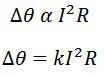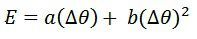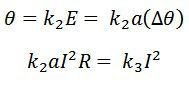# Thermocouple Instrument

Definition: The instrument which uses the thermocouple for the measurement of the temperature, current and voltage such type of instrument is known as the thermocouple instrument. It is used for both the AC and DC measurement.

The thermocouple is an electric device which uses two wires of the different metal. It’s work on the principle that the heat energy is converted into an electric energy at the junction which is made of different metals.The current whose magnitude is used to be measured passes through the junction of the thermocouple. The current produces the heat in the heater element, and the thermocouple induces the electromotive force in the output terminals. This emf is measured through the PMMC instrument. The magnitude of the emf is proportional to the temperature and the RMS value of the current.

The most significant advantage of the thermocouple instrument is that it is used for high-frequency measurement of current and voltage. The instrument is more accurate for the frequency above 50Hz.

## Principle of Operation of Thermoelectric Instrument

The circuit in which thermal emf generates is made of two dissimilar metals. The temperature at their junction is written asWhere the a and b are constant, and their value depends on the properties of metal used in the thermocouple. The approximate value of the a is 40 to 50 microvolt, and that of the b is a few tenths to hundreds of microvolt/C2.

Let = Δθ temperature difference between hot and cold junction. Thus their temperature is expressed asThe heater produces the heat which is directly proportional to the I2R. Where I is the RMS value of current and R is the resistance of the heating element. The rise in temperature is proportional to the heat produces by the heating element.The thermocouple instrument has two junctions cold and hot. The difference between these two junctions is expressed asThe value of b is very small as compared to the a and hence it is neglected. The temperature at the junction is expressed asThe deflection of PMMC instrument is directly proportional to the emf induces in the terminals. The deflection of the moving coil instruments is expressed asWhere K3 – aK1K2R = a constant.

The instrument shows the square law response.

## Construction of Thermoelectric Instrument

Thermoelectric element and the indicating instrument are the two main parts of the thermo-electric instrument.

### Thermoelectric elements

Four types of thermoelectric element are used in the thermocouple instrument. Their types are explained below in details.

1. Contact Type – The contact type element uses the separate heater. The junction of the thermocouple is contacted with the heater shown in the figure below.The electric heating element is used for the following purposes.

1. The heater element converts the electrical energy into thermal energy.
2. The heat energy is converted into thermal energy with the help of Seebeck effect.
3. The output terminal of the thermocouple is connected to PMMC instrument. The smallest amount of the electric energy is used for deflecting the pointer of PMMC instrument, and this energy is stored in the spring of the instrument.

2. Non-contact type Instrument – In this instrument, there is no electrical contact between the heating element and the thermocouple. It is separated by the electrical insulation. The insulation makes the system less sensitive and slow as compared to the contact type instrument.

3. Vacuum Thermo-Element – In the vacuum tube instrument, the heater and the thermocouple are placed in the evacuated glass tube. This vacuum tube increases the efficiency of the instruments because heater remains hot for a long time.4. Bridge Type – In the bridge type instrument, the current is directly passed through the thermocouple and raises their temperature. The temperature of the thermocouple is directly proportional to the RMS value of current.The following are the advantages of thermoelectric Instrument.

1. It directly indicates the RMS value of voltage and current on the waveform.
2. Such type of instrument is free from the stray magnetic field.
3. The thermic electric element is used for a wide range of current measurement.
4. The instrument has a high sensitivity.
5. It is useful for the calibration of the potentiometer with the help of the standard cell.
6. The thermic element is free from the frequency error and hence used for the widest range of frequency.